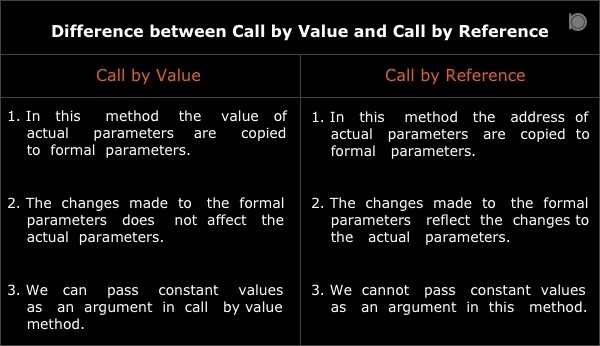C language has two techniques by which we can pass arguments to the function.

1. Call by Value
2. Call by Reference

## 1. Call by Value

In call by value method the values of actual parameters are copied to the formal parameters. These formal parameters are also called as dummy parameters. In this method the changes made to formal parameters doesnot affect the actual parameters.

### Program to swap two numbers using call by value method

``````/* Program to swap two numbers using call by value method */
#include<stdio.h>

int main()
{
int a = 2, b = 3;
void swap(int, int);

printf("Value of a and b before swapping is %d and %d\n", a, b);

swap(a, b);

printf("Value of a and b after swapping is %d and %d\n", a, b);
return 0;
}

void swap(int x, int y)
{
int z;
z = x;
x = y;
y = z;
}
``````

### output

``````Value of a and b before swapping is 2 and 3
Value of a and b after swapping is 2 and 3
``````

In the above example we saw that even if we had swapped the value of formal parameters there is no effect of it on the actual parameters.

## 2. Call by Reference

In call by reference method the address of the actual parameters are copied to the formal parameters instead of copying the actual value. In this method changes made to the formal parameters directly affect the actual parameters. To pass the actual parameters as reference we need to declare formal parameter as pointer types.

### Program to swap two numbers using call by reference method

``````/* Program to swap two numbers using call by reference method */
#include<stdio.h>

int main()
{
int a = 2, b = 3;
void swap(int*, int*);

printf("Value of a and b before swapping is %d and %d\n", a, b);

swap(&a, &b);

printf("Value of a and b after swapping is %d and %d\n", a, b);
return 0;
}

void swap(int *x, int *y)
{
int z;
z = *x;
*x = *y;
*y = z;
}
``````

### output

``````Value of a and b before swapping is 2 and 3
Value of a and b after swapping is 3 and 2
``````

In the above example we have seen that even the changes made to the formal parameters are reflected back to the actual parameters.

## Difference between Call by Value and Call by Reference